# Distortion

## Characterization

Lens distortions in an imaging system are a result of a camera's lens assembly not perfectly representing the pinhole camera model that we use to relate our world (object space) to our image coordinate system. Lens distortions can be broken into roughly three effects:

1. Symmetric radial distortion models a kind of distortion (such as barrel or pincushion distortion) that applies across the image extent radially and is irrespective of the distance of any objects in the scene.
2. Tangential or decentering distortion models non-orthogonalities (decentering) in the lens-assembly of a camera, and quantifies how that deviates from a pinhole model. As decentering errors in the lens-assembly gets smaller, these errors can often correlate highly with small errors in $$c_x$$ and $$c_y$$. As a result, these effects sometimes go uncharacterized as their observability can be low.
3. Asymmetric radial distortion comprises effects in the radial direction of our observations that are dependent on the relative distances between objects in the scene. In many cases, asymmetric radial distortion effects are modeling refraction of light rays in our object space or "world," and not necessarily lens effects. As a result, these effects are difficult to observe in close-range applications, and we often choose not to model them so as not to over-fit our model.

Most models operate as a function of the distance to the principal point of the lens, modeled by r, as well as angles between the principal point and image space.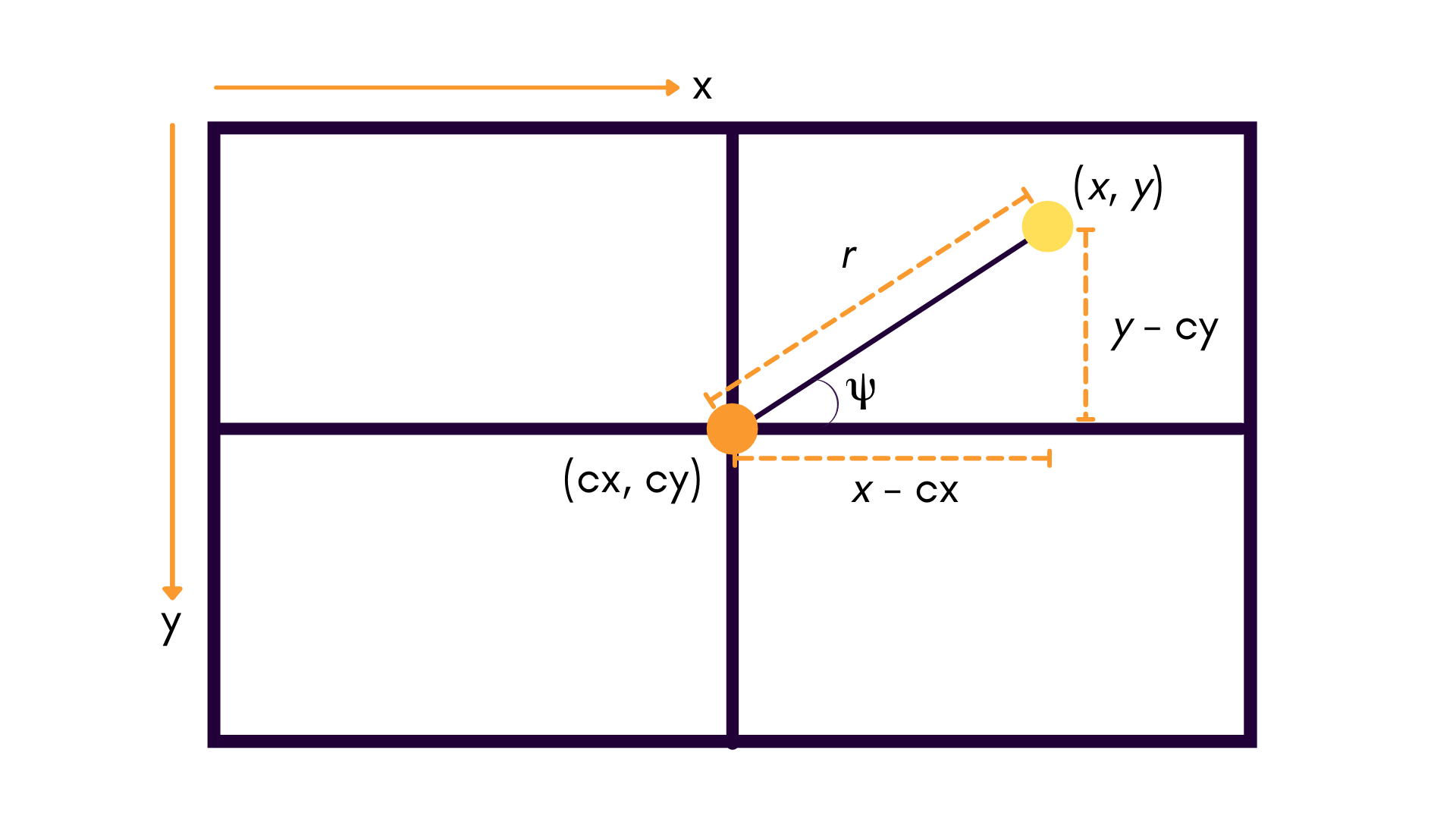We've written in-depth about camera distortions on the Tangram Vision Blog. See Part 1 and Part 2 of our distortion series for more information.

## Supported Distortion Models

Brown-Conrady is the typical distortion model used by default in most calibration pipelines. With it, we can model both the symmetric radial distortion and the tangential distortion of the lens:

$r = \sqrt{(x - c_x)^2 + (y - c_y)^2}$
$\Delta r_{\mathsf{symm\ rad}} = k_1 r^3 + k_2 r^5 + k_3 r^7$
$\Delta x_{\mathsf{tangential}} = p_1 (r^2 + 2 (x - c_x)^2) + 2 p_2 (x - c_x)(y - c_y)$
$\Delta y_{\mathsf{tangential}} = p_2 (r^2 + 2 (y - c_y)^2) + 2 p_1 (x - c_x)(y - c_y)$

Note

Radial distortion terms can expand beyond $$k_3$$. This would just mean that there are major distortion artifacts near the edges of the field of view. However, This is not usually done in practice; most lenses are manufactured well nowadays, and more terms aren't necessarily needed for an accurate model.

Based on these definitions, the radial distortion terms are often simplified into $$x$$ and $$y$$ components:

$cos(\psi) = \frac{(x - c_x)}{r}$
$sin(\psi) = \frac{(y - c_y)}{r}$
$\Delta x_{\mathsf{symm\ rad}} = (x - c_x) (k_1 r^2 + k_2 r^4 + k_3 r^6)$
$\Delta y_{\mathsf{symm\ rad}} = (y - c_y) (k_1 r^2 + k_2 r^4 + k_3 r^6)$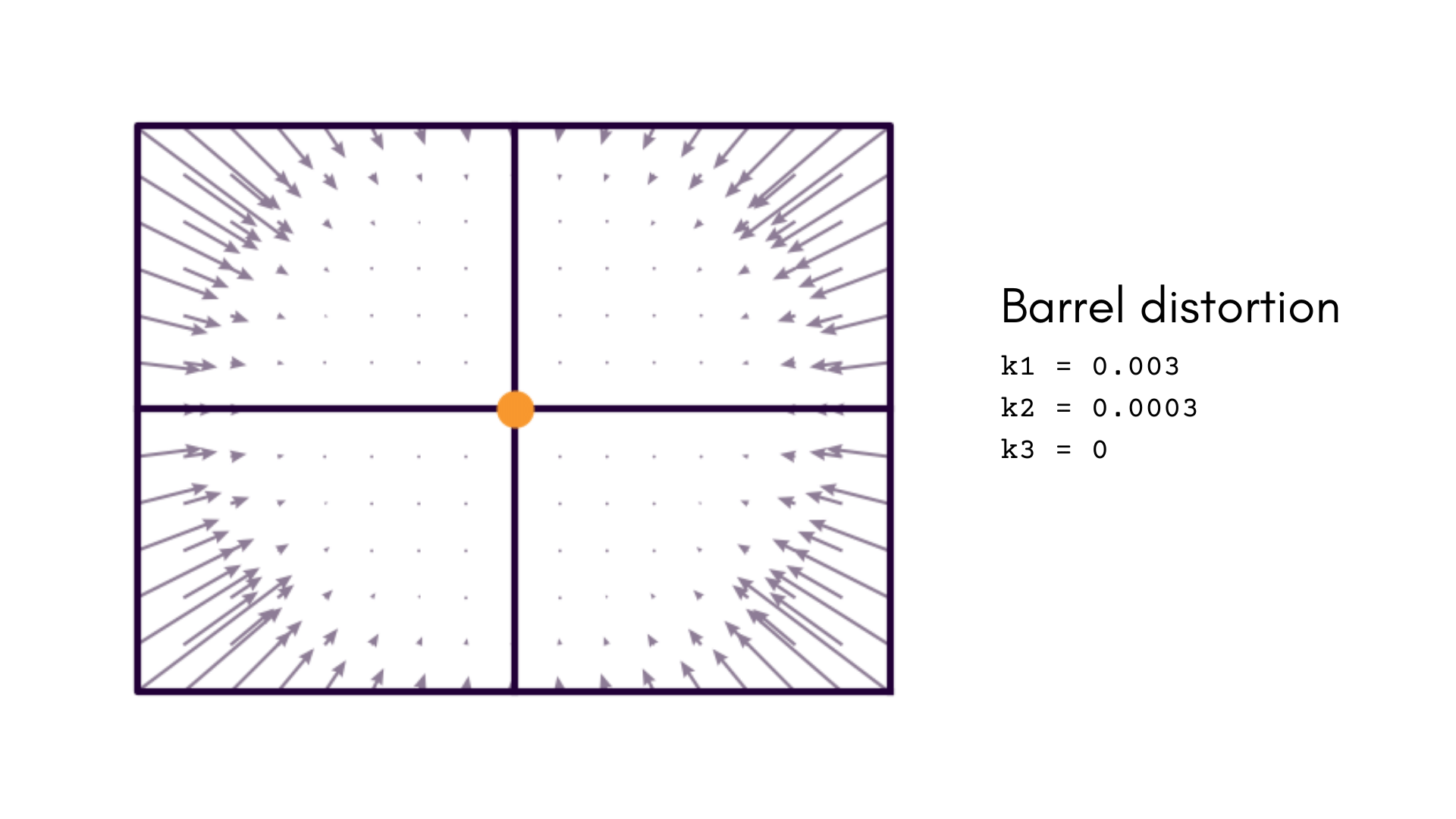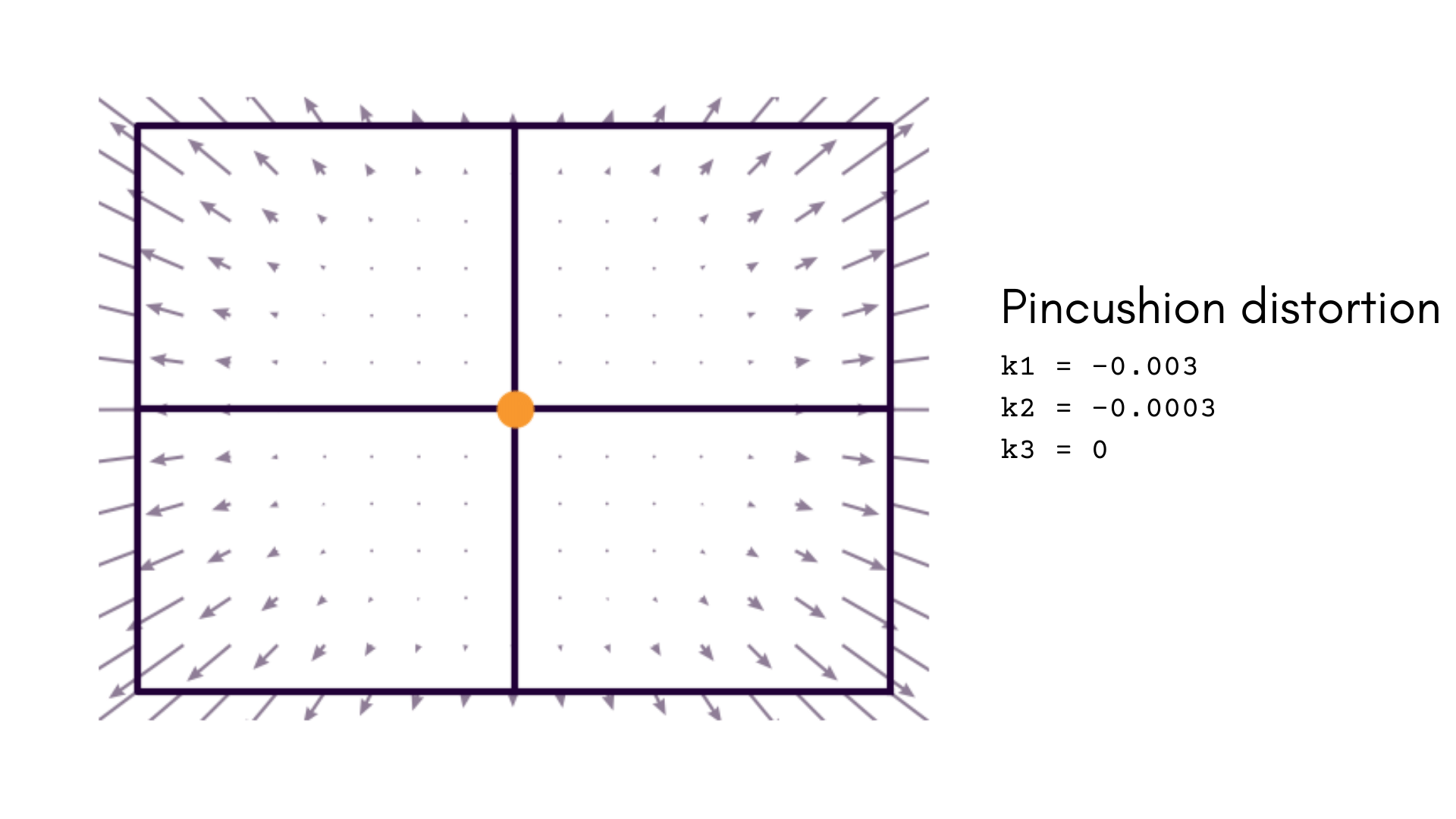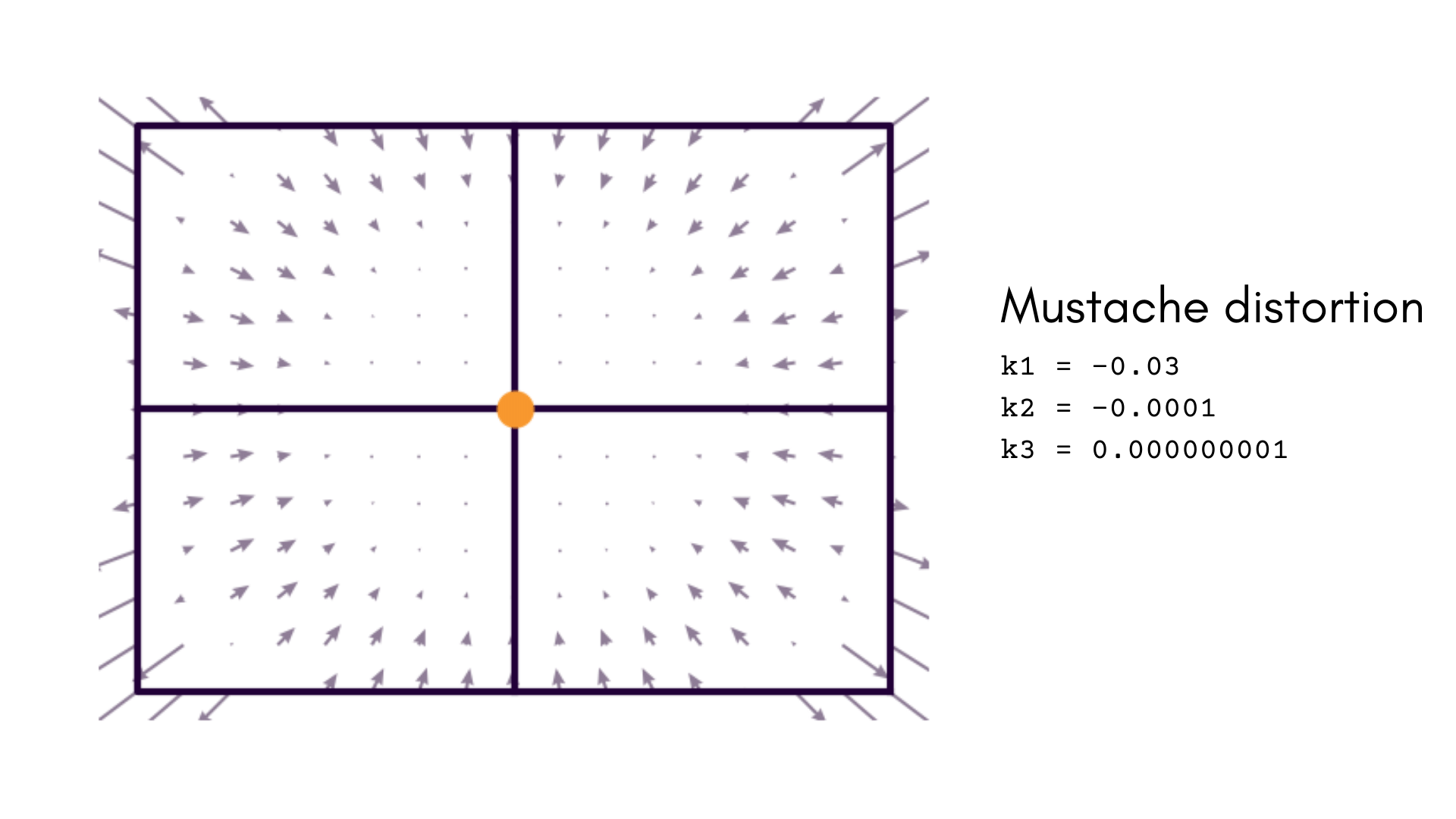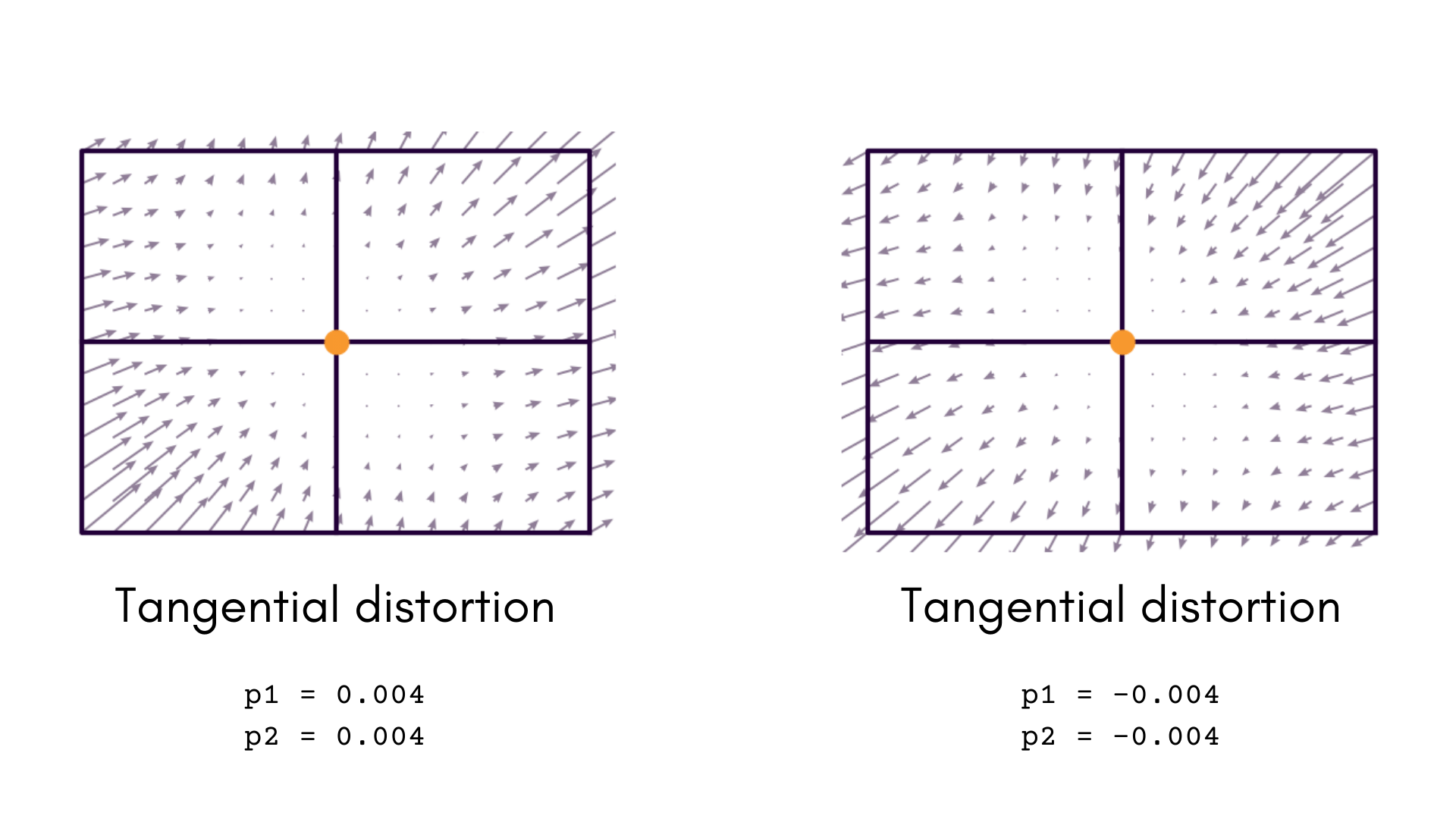The Brown-Conrady distortion model is useful for cameras that have a standard field-of-view lens. The tangential components of this distortion can often correlate with $$c_x$$ and $$c_y$$, and so may be left out. However, in cameras with complex lens assemblies (i.e. a compound lens with many parts), decentering distortion is very likely to be a problem and should be measured.

The Brown-Conrady distortion model is originally described in two papers. Read them here and here.

### Kannala-Brandt

The Kannala-Brandt model is an empirically derived model that is parameterized slightly differently than Brown-Conrady. Instead of parameterizing the radial distortion according to the radial component of the polar image point $$r$$, it is instead parameterized in terms of $$\theta$$, the angle between the principal axis and an extended ray to the image point.

The Kannala-Brandt distortion model is most useful when used with high field-of-view lenses (typically greater than 90° FOV). Smaller field-of-view lenses are better served by the Brown-Conrady model, as it is conceptually simpler and tends to reduce projective compensation.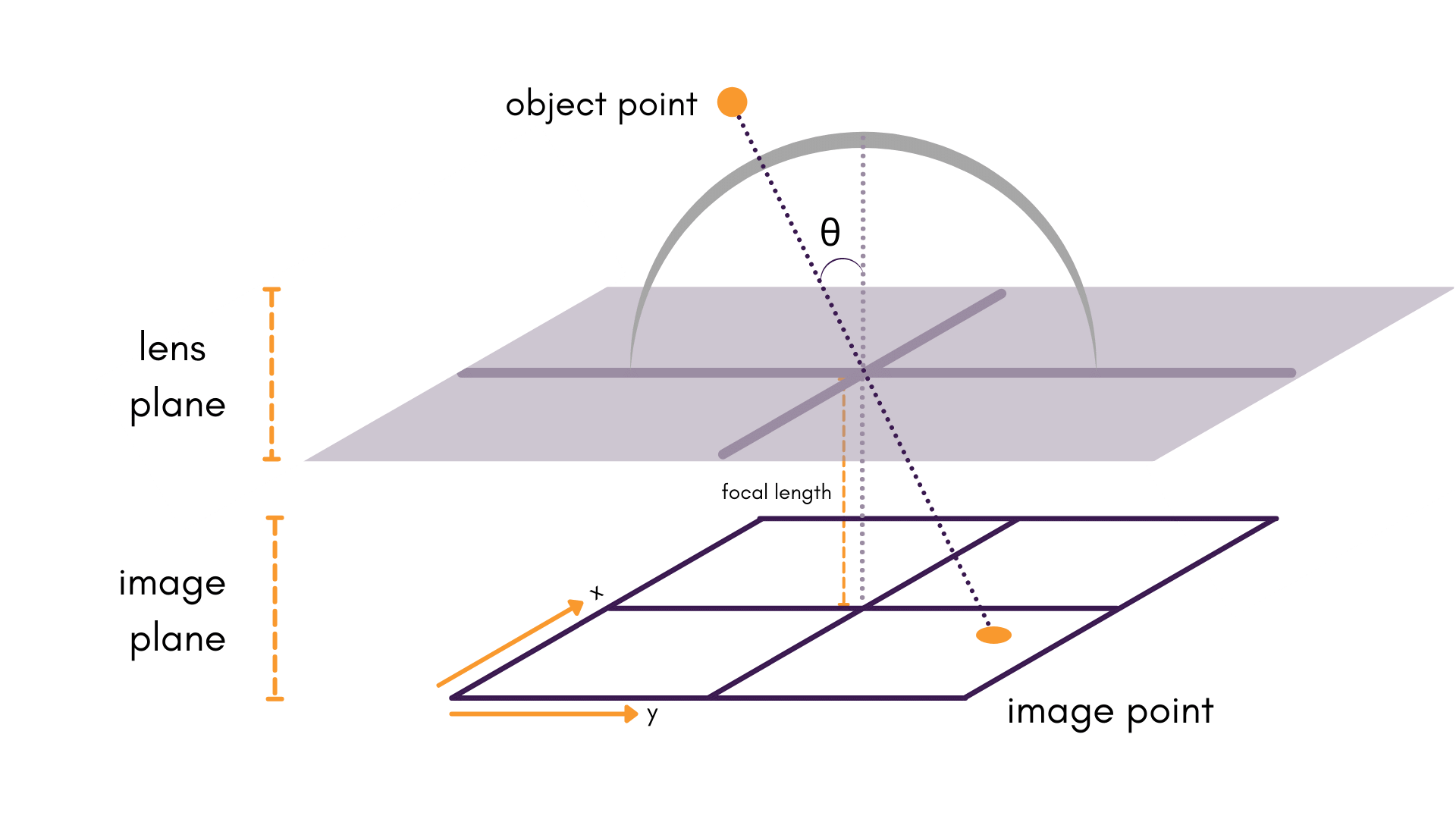The complete Kannala-Brandt model contains separate terms for all three distortion types; however, the Tangram Vision Platform only supports symmetric distortion. Thus, the Kannala-Brandt model can be summarized as:

$\Delta r_{\mathsf{symm\ rad}} = k_1 \theta + k_2 \theta^3 + k_3 \theta^5 + k_4 \theta^7$

Note

Only the first four terms of this expansion are modeled. Symmetric terms beyond $$k_4$$ are seldom used and are typically insignificant.

As with Brown-Conrady, this is often broken into:

$\Delta x_{\mathsf{symm\ rad}} = \frac{(x - c_x)}{r} (k_1 \theta + k_2 \theta^3 + k_3 \theta^5 + k_4 \theta^7)$
$\Delta y_{\mathsf{symm\ rad}} = \frac{(y - c_y)}{r} (k_1 \theta + k_2 \theta^3 + k_3 \theta^5 + k_4 \theta^7)$